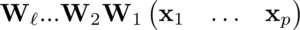Machine Intelligence

# Matrices in machine learningThis article gives a sense of how matrix operations such as matrix multiplication arise in machine learning.

# Dense layers

Before we get started, there is a small bit of prerequisite knowledge about “dense layers” of neural networks.

1. What is a “dense layer”? Don’t worry about how “dense layers” fit into the big picture of a neural network. All we need to know is that a dense layer can be thought of as a collection of “nodes”.

2. Well now we’ve begged the question: what is a “node”? A node can be thought of as a machine, associated with a set of numbers called weights, that is responsible for producing a numeric output in response to receiving some number of binary (i.e. 0 or 1) inputs. Specifically, if a node N is associated with n weights w1, …, wn, then, when n binary numbers x1, …, xn are given to N as input, N will compute the following “weighted sum” as output:For example, if a node has weights 3, 4, 5 and is passed the binary inputs 1, 0, 1, then that node will return the weighted sum 3 * 1 + 4 * 0 + 5 * 1 = 8.

That’s it! We are done with covering prerequisites. We now know what a dense layer of a neural network is responsible for accomplishing: a dense layer contains nodes, which are associated with weights; the nodes use the weights to compute weighted sums when they receive binary inputs.

# Matrices

Now, we see what happens when we consider all of the nodes in a dense layer producing their outputs at once.

Suppose L is a dense layer. Then L consists of m nodes, N1, …, Nm for some whole number m, where the ith node Ni has n weights* associated with it, wi1, …, win. When Ni receives n binary (0 or 1) inputs x1, …, xn, it computesSince the above weighted sum is computed by the ith node, let’s refer to it as f(Ni):The nodes N1, …, Nm will need to compute the weighted sums f(N1), …, f(Nm):The above can be rewritten with use of so-called column vectors:A column vector is simply a list of numbers written out in a column. Note that in the above we have made use of the notions of “column vector addition” and “scaling of a column vector by a number”.

The above expression can be expressed as a matrix-vector product:(The matrix-vector product Wx of the above is literally defined to be the expression on the right side of the previous equation).

This matrix-vector product notation gives us a succinct way to determine what each node in a layer does to inputs x1, …, xn.

## Generalization

The above approach generalizes in the following way. Assume that instead of n inputs x1, …, xn, we have p groups of inputs, where the ith group has inputs x1i, …, xni. Additionally, define xi := (x1i, …, xni) and let f(Nj, xi) denote the result of node Nj acting on x1i, …, xni. Then we haveThis is equivalent to## Further generalization

Now, assume that in addition to having multiple groups of inputs x1, …, xp, we want to pass these groups of inputs through multiple layers multiple layers L1, …, Ll. If layer Li has weight matrix wi, then the result of passing x1, …, xp through L1, …, Ll is the following product of matrices:* You may object and remark that the number of weights associated with a given node depends on the node; in other words, that node each node Ni has ni weights associated with it, not n weights. We are justified in assuming that ni = n for all i because we can always associate extra weights of 0 to nodes that don’t already have the requisite n weights associated with them.

This site uses Akismet to reduce spam. Learn how your comment data is processed.

##### Ross Grogan-Kaylor

Ross Grogan-Kaylor is a Technical Consultant at Perficient’s Minneapolis office. He enjoys engaging with structural patterns in the syntax and in the high-level ideas of software development.

More from this Author